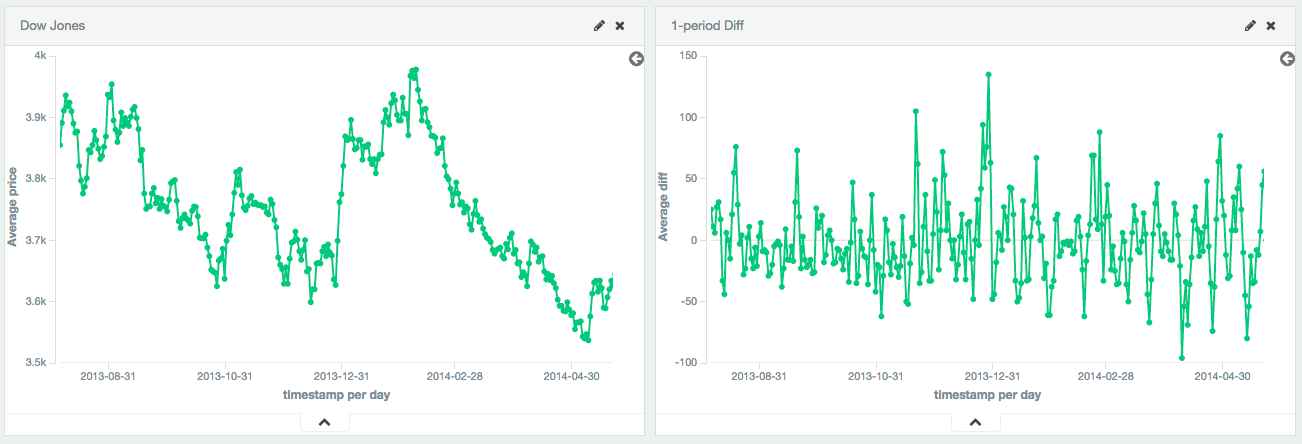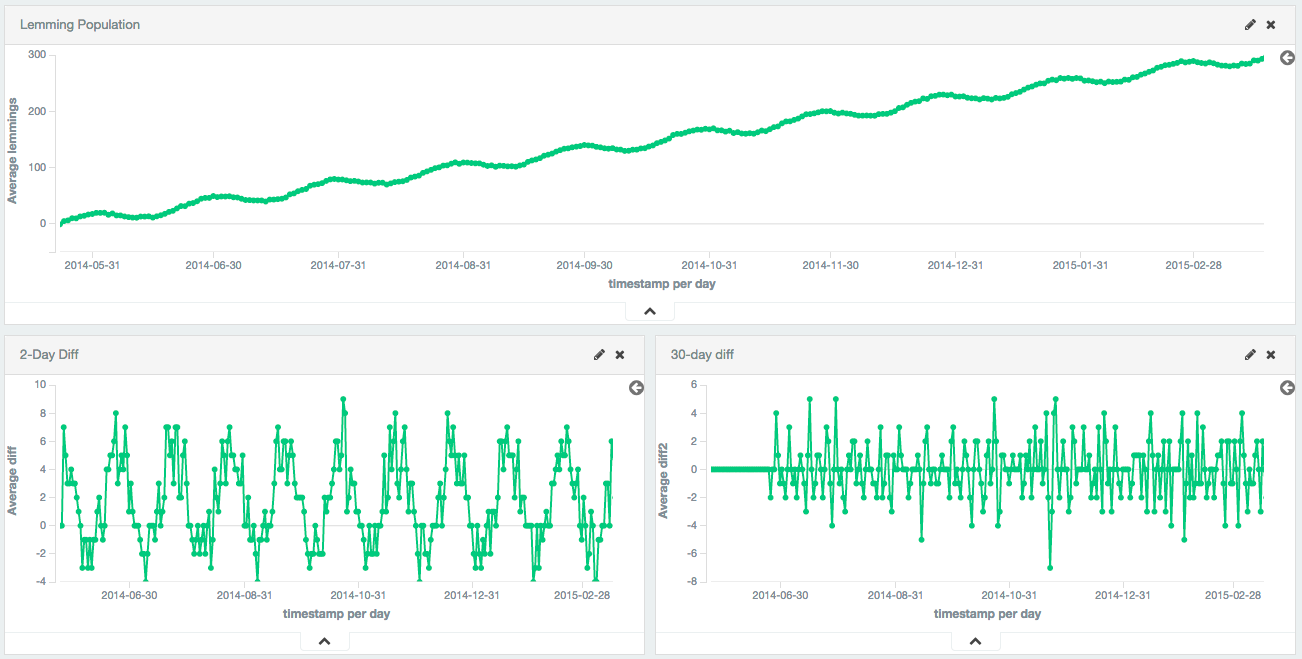## Serial differencing aggregationedit

Serial differencing is a technique where values in a time series are subtracted from itself at different time lags or periods. For example, the datapoint f(x) = f(xt) - f(xt-n), where n is the period being used.

A period of 1 is equivalent to a derivative with no time normalization: it is simply the change from one point to the next. Single periods are useful for removing constant, linear trends.

Single periods are also useful for transforming data into a stationary series. In this example, the Dow Jones is plotted over ~250 days. The raw data is not stationary, which would make it difficult to use with some techniques.

By calculating the first-difference, we de-trend the data (e.g. remove a constant, linear trend). We can see that the data becomes a stationary series (e.g. the first difference is randomly distributed around zero, and doesn’t seem to exhibit any pattern/behavior). The transformation reveals that the dataset is following a random-walk; the value is the previous value +/- a random amount. This insight allows selection of further tools for analysis.Figure 3. Dow Jones plotted and made stationary with first-differencing

Larger periods can be used to remove seasonal / cyclic behavior. In this example, a population of lemmings was synthetically generated with a sine wave + constant linear trend + random noise. The sine wave has a period of 30 days.

The first-difference removes the constant trend, leaving just a sine wave. The 30th-difference is then applied to the first-difference to remove the cyclic behavior, leaving a stationary series which is amenable to other analysis.Figure 4. Lemmings data plotted made stationary with 1st and 30th difference

### Syntaxedit

A `serial_diff` aggregation looks like this in isolation:

```{
"serial_diff": {
"buckets_path": "the_sum",
"lag": 7
}
}```

Table 72. `serial_diff` Parameters

Parameter Name Description Required Default Value

`buckets_path`

Path to the metric of interest (see `buckets_path` Syntax for more details

Required

`lag`

The historical bucket to subtract from the current value. E.g. a lag of 7 will subtract the current value from the value 7 buckets ago. Must be a positive, non-zero integer

Optional

`1`

`gap_policy`

Determines what should happen when a gap in the data is encountered.

Optional

`insert_zeros`

`format`

DecimalFormat pattern for the output value. If specified, the formatted value is returned in the aggregation’s `value_as_string` property

Optional

`null`

`serial_diff` aggregations must be embedded inside of a `histogram` or `date_histogram` aggregation:

```POST /_search
{
"size": 0,
"aggs": {
"my_date_histo": {
"date_histogram": {
"field": "timestamp",
"calendar_interval": "day"
},
"aggs": {
"the_sum": {
"sum": {
"field": "lemmings"
}
},
"thirtieth_difference": {
"serial_diff": {
"buckets_path": "the_sum",
"lag" : 30
}
}
}
}
}
}```
 A `date_histogram` named "my_date_histo" is constructed on the "timestamp" field, with one-day intervals A `sum` metric is used to calculate the sum of a field. This could be any metric (sum, min, max, etc) Finally, we specify a `serial_diff` aggregation which uses "the_sum" metric as its input.

Serial differences are built by first specifying a `histogram` or `date_histogram` over a field. You can then optionally add normal metrics, such as a `sum`, inside of that histogram. Finally, the `serial_diff` is embedded inside the histogram. The `buckets_path` parameter is then used to "point" at one of the sibling metrics inside of the histogram (see `buckets_path` Syntax for a description of the syntax for `buckets_path`.